Lecture 11
Chi-square

Chi-square (or X2 after the Greek letter for c) is a widely used statistical test which is officially known as the Pearson chi-square in homage to its inventor, Karl Pearson. One reason it is widely used is that it can help answer a number of different types of analytic questions. Daniel discusses a several of these different uses. Despite all of these potential uses, probably 90% of all uses of chi-square involve analysis of contingency tables covered on pp. 595-598. A 2 X 2 contingency table is a table that presents counts of participants in each of four cells. The four cells are formed by two dichotomous variables. The contingency table analysis is the only chi-square test that we will calculate in this class, but it is important for you to understand the other uses of chi-square. So, I'll devote this lecture to contingency table analysis and then mention some of the other chi-square uses.

Chi-square can be thought of in several ways. The first way we can think of the chi-square test is as an analogy to the t-test in which we are interested in comparing two groups. Only chi-square is used instead, because the dependent variable is dichotomous. So, a 2 X 2 ("two-by-two") chi-square is used when there are two levels of the independent variable and two levels of the dependent variable. This might be called a test of homegeneity because we are testing whether two groups are the same. Homegeneous means "same type."

Another way to think of the same test is as a goodness-of-fit test. The goodness-of-fit test concerns frequencies of participants in a sample, and whether or not those frequencies are the frequency we would expect due to chance. For instance, the frequencies might involve how many participants said "no" and "yes" in response to a survey question. Imagine a survey question in English that was posed to an immigrant population that did not understand English well. If the respondents to the survey did not understand the question asked, frequency of no and yes responses might be a matter of chance. The goodness-of-fit analytic question then concerns whether or not the survey respondents said yes or no with a frequency that we would expect due to chance or not. If the respondents did not understand the question, we would expect about 50% to answer yes and 50% to answer no. The goodness-of-fit, then concerns whether the actual frequency of yes/no responses matches the frequency we would expect due to chance.

Now, it turns out that the question of homogeneity (group differences) and question of goodness-of-fit are really the same question. If we ask whether two groups (say males and females) are equal in their tendency to say yes to a survey question, we are also asking whether the rate at which each group responds yes and no is the rate we would expect from chance. Thus, the computation of chi-square concerns the match between the expected frequencies, based on the overall number of people in each group, and the obtained frequencies.

Chi-square can also be thought of as a test of whether two variables are independent or not, and, thus, it is considered a test of independence. An independence question might concern whether gender (male vs. female) is related to responses to a yes/no question of a survey. If gender is related to saying yes to the question, the two variables are not independent of one another--they are dependent or related.

So, researchers use chi-square to answer questions about homogeneity, goodness-of-fit, or independence. If you think hard enough about these three questions, they all turn about to be the same question! Heavy, huh?!

Contingency tables (2 X 2)
The contingency table is just a summary table of the data. Let's take an example of a typical use of chi-square that compares two groups on some dichotmous measure. Let's say we accessed some local data on voter registration to see if males and females were equally likely to be Republican or Democrat. We got a list of 180 voters and entered their political affiliation into a database. To do that we just need two variables (other than an ID variable)--gender and political affiliation. We would enter that data in the normal way (using codes 0 and 1 to indicate male and female etc.), but, for convenience, we (or a computer) would summarize that information in a table like this:

 Females Males Total Democrats 50 70 120 Republicans 19 41 60 Total 69 111 180

There are 180 cases total, with 120 Democrats, 60 Republicans, 69 females, and 111 males. Overall, there are more males than females, so to know if males or females are more likely to be Democrat or Republican, we need to take into account the total number of each. Because there are more males, we expect there to be a higher frequency of males in both the Republican and Democratic groups. So, we need to take those base rates into account to figure out the frequencies we would expect due to chance.

The formula for computing chi-square is a matter of gauging how closely our observed frequencies in each cell of the table are matched to the expected frequencies.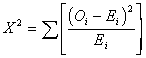In the table Oi stands for the observed frequency in one cell and Ei stands for the expected frequency of that cell. As you can see in the formula, we compute chi-square by summing up the differences between the observed and the expected frequency for each cell.

When we do not have any information about the expected frequency in each cell, we can compute it based on the total number of males and females or yes and no responses. As long as we are testing the notion that the cells are equal or not in their expected rate, we can use the following handy-dandy computation formula instead of going through the involved process of calculating the expected frequencies, computing the differences, and summing them up. Here is the short hand method: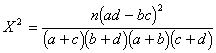This is much easier than it looks, because a, b, c, and d just refer to the frequency in each of the cells. n, as usual, is the number of cases in the sample. The formula does not make too much sense, because it is just a short cut for computing chi-square when we have a 2 X 2 contingency table.

 Females Males Democrats a b Republicans c d

We just do some muliplying, adding, subtracting, and squaring and, presto, a chi-square. Here we go: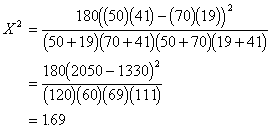To see if this descrepancy between observed and expected is likely to be due to sampling error, we test the calculated chi-square for significance. The d.f. is equal to (r - 1)(c - 1) = (2 - 1)(2 - 1) = 1, where are r is the number of rows and c is the number of columns. We look up in Table F in the back of the book under the .95 column (for alpha = .05). The critical value in the table is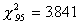, and we conclude that there are no significant differences between males and females in their political affiliations.

More than 2 categories
Chi-square can also be used with more than two categories. For instance, we might examine gender and political affiliation with 3 categories for political affiliation (Democrat, Republican, and Independent) or 4 categories (Democratic, Republican, Independent, and Green Party). In that case we can't use our handy-dandy formula for 2 X 2 tables, but we can use a computer! Because chi-square can be thought of as a test of goodness-of-fit, it doesn't really matter whether we think of gender or political affiliation as the independent variable. The test will be the same either way. Chi-square then applies to situations where we have 2 X 3 or 3 X 4 or 5 X 6 tables. When there is more than a 2 X 2 table, we have an omnibus test, because the test just indicates if there is any differences among the cells. To look for specific cell differences, researchers usually analyze separate subtables of 2 X 2s or another method called loglinear analysis (which we won't cover in the class).

Other measures of fit
You will also see some alternatives to Pearson chi-square. Two, in particular, are the likelihood ratio test (G2) and the Neyman weighted least squares chi-square (Q). We will discuss the likelihood ratio test more later, and the Neyman test is not used very often. All three are very similar, especially with a large sample size.

Yate's Correction and Fisher's Exact Test
You will often see two other statistics printed out by statistical packages. Yate's continuity correction is a correction of Pearson chi-square that adjusts the chi-square for smaller samples. The reason for the correction is that the sample is based on dichotomous data, and critical chi-square values are based on a continuous distribution. Incidentally,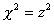or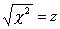, so chi-square is a function of the normal distribution (remember the end of the lecture on the
binomial distribution?). Yates correction is usually a conservative estimate of chi-square, because the value of chi-square is adjusted downward. There is considerable disagreement about whether or not Yate's correction is too conservative or not, and judging from most stats texts I've seen, I think most statisticians prefer not to use it.

The Fisher's exact test is used when there are unequal numbers in the groups, and the accuracy of the test is a concern, because there are very low expected frequencies in the cells. Fisher's exact test adjusts chi-square for this problem. Usually, Pearson chi-square is ok if there is an expected frequency of 5 or more in 80% of the cells, and an expected frequency of 1 or more in the remaining 20% of cells. Computer packages usually print a warning when these expected frequencies are low. When this warning occurs, many researchers like to examine the Fisher's exact test.

Non-contingency Analysis with Chi-square
Above I mentioned that the chi-square calculations I used were based on the assumption that by chance, we expect that there is an equal probability of occurrence in each group. For instance, chance of responding yes or no is 50% for each. Usually the expected frequency is not known and must be computed from the data, and the assumption is that there will be equal proportions (e.g., 50% for 2 groups, 33% for three groups, and so on). Sometimes, however, the proportion expected from chance is known by the researcher or there is some reason to expect what the distribution will be like. In this case, the Poisson distribution or another distribution can be used to compute the expected frequencies. These other situations are discussed more completely by Daniel. I think you should review them and understand when they are used, but, because they are seen relatively infrequently, I do not want you to spend a lot of time on them. When you run into these situations, the Daniel text will provide a nice reference book if you need to figure out how to calculate these or whether your situation is relevant.